# Lesson 6

## Introduction

#### Course Objective

Learn to project the total population size of a district, city, town, and rural area.

#### Expected Outcome

Ability to use several tools to project the population size of a given locale.

This lesson presents several simple projection tools that can be used to determine future demand for basic services such as safe water, fire and police protection, and electricity and health care services for a total population. These tools are helpful in situations where past and current census data are used to project the population size of a future date. It is possible to use these tools to project a sub-set of the population, such as the number of children under the age of 15 for a 5-year education plan.

Two types of projection tools are explored: extrapolation methods and prorating tools. Discussions of both tools will include basic assumptions, indications for use, and examples for use with census data. The projection tools do not account for deaths due to AIDS, as it is difficult to estimate or project the number of AIDS deaths for sub-areas of a country.

### 6.1 Extrapolating the Population into the Future

Extrapolation is a projection technique that uses aggregated data from the past to project into the future. In population projections, past and current census information is used to project future population size. In most cases, extrapolation methods are used for projecting the total population size for a future date in time. However, these tools can also be used to project the size of population segments, provided that both past and current census data are available.

Basic Assumption
The characteristics of the more recent periods of development for the locale are expected to continue into the future.
Use These Tools
• to determine the total size of the population for a future date
• when data availability is limited to past and current census information
• when the time period is relatively short; i.e., 5 to 10 years into the future
• when past growth of the locale is relatively stable; i.e., there have been no sharp increases or declines when examining past census takings

This section introduces several extrapolation methods. The process of selecting an appropriate extrapolation tool is described below.

1. Obtain as much past census information as possible for the given locale. Plot the population size for each census taking on graph paper, with population on the vertical line (y-axis) and time on the horizontal line (x-axis), as shown in Figure 6-1.

Figure 6-1
Plot of population versus time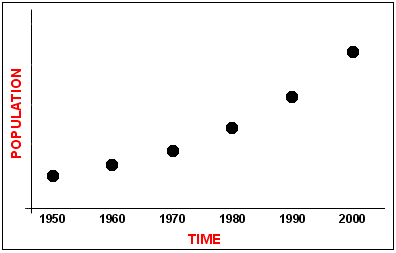1. Draw lines to connect the census points on the diagram.
2. Based on the drawing, select an extrapolation tool that describes the population trends of the locale and best meets the assumptions of the tool.
3. Apply an extrapolation tool and project the population for a date in the future.
4. Compare the results with another projection.

The discussion of extrapolation tools presented in the following section relies on three classic references: Krueckeberg and Silvers (1974); Pittenger (1976); and Croxton, Cowden, and Klein (1967). Three extrapolation tools will be introduced: the linear, exponential, and the modified exponential models.

The Linear Model

The linear model, when plotted on a graph, takes the form of a straight line as shown in Figure 6-2.

Figure 6-2
Linear Population Growth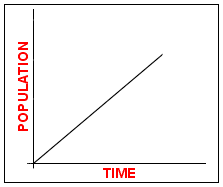Assumption:
The linear model assumes that population growth is growing at absolute equal increments per year, decade, or other unit of time. It also assumes that growth will follow a similar pattern in future years.
When best to use:
Use when the pattern of growth is similar to a straight line. This tool is especially useful when projecting areas experiencing slow growth.

To test the model, graph the data to see if growth is linear. In addition, calculate the differences in absolute growth to determine if there are equal differences (see Figure 6-3). The last assumption is difficult to meet with census data because locales do not experience equal population growth between each census taking.

If the growth pattern on the graph displays a linear form, there are three options.

1. Use Equation 6-1 (shown below) if the model is the best fit of the census data
2. Use a ruler and extend the line into the future
3. Use linear regression analysis, an advanced statistical tool, when the pattern of growth appears to be linear and growth is unequal between census takings. In regression analysis, a line goes through the data points (dots of the plotted census data) that minimize the sum of squared vertical distances or deviations from the line. The census data must meet the assumptions of linear regression as follows:
1. X and Y must have a linear relationship
2. residuals are independently distributed with a mean of 0
3. the variance of Y is constant over all values of X

If necessary, review a basic statistics book prior to using linear regression analysis.

Equation 6-1
The Linear Equation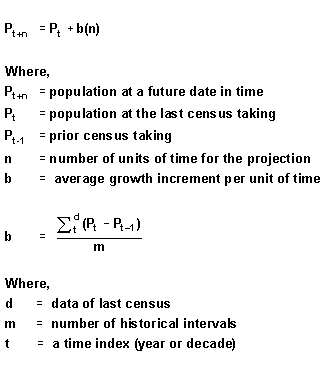All three approaches are used in the following example.

Example Using an Urban County: Durham County, North Carolina

The basic assumption of the linear model is that population growth takes place at equal increments per year or unit of time. This assumption is impossible to meet with census data. The second assumption is that growth takes on a linear pattern.

To determine whether or not the linear model can be used, use census data for Durham County to plot population versus time to evaluate if the relationship is linear. Figure 6-3 presents a graph of census data for the county from 1960-2000.

Figure 6-3:
Durham County, North Carolina 1960-2000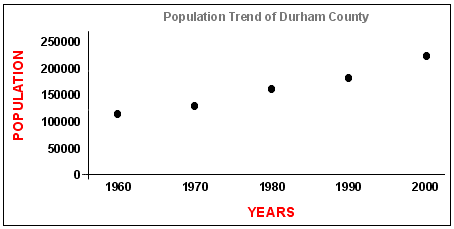Examine Figure 6-3. Can the linear model be used? It is a difficult decision. The form of the line is almost linear. In addition, Durham County appears to be experiencing slow growth. Since the pattern of growth appears to be linear, all three linear models can be applied. The three can then be compared to determine which comes closest to providing an accurate projection.

Linear Approach 1: Use of a Ruler
Result: Year 2010 = 266,667

Step 1: Plot the census data from 1960-2000 plotted on lined graph paper

Step 2: Use a ruler to extend the line from 1990 and 2000 to the projection year of 2010.

The projected population size for Durham County of 2010 is 266,667.

Linear Approach 2: Use of the Linear Equation
Result: Year 2010 = 251,143

Using the linear equation (presented in Equation 6-1) with Durham County data, results indicate that equal growth is not taking place among census periods, especially for the last census period (see data table in Figure 6-3). The result raises questions about using the linear equation for this projection. The calculation is shown in the example below.

Example of Equation 6-1
Use of the Linear Equation for Durham County, 1960-2000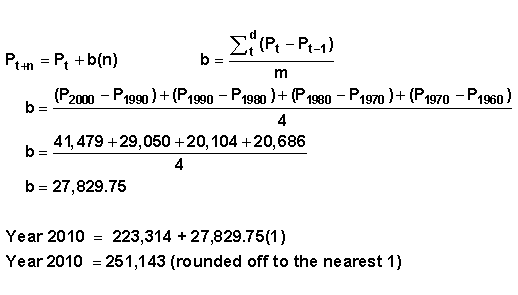Linear Approach 3: Use of Linear Regression Analysis
Result: Year 2010 = 242,060

In linear regression analysis, a line goes through a series of data points. As mentioned earlier, linear regression analysis can be used when the growth pattern appears to be linear and population growth is unequal between census takings. The linear regression equation is shown in Equation 6-2. The equation is similar to the one used for the linear equation. The calculation process, however, is different.

Equation 6-2
The Linear Regression Equation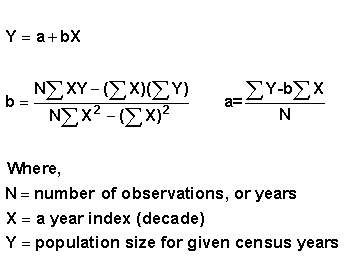The next section demonstrates how to set up the information for the linear regression equation. Note that the forecast tool in Microsoft Excel can perform the linear regression and will produce the same results in minutes.

 Table 6-1Preparing the Table to Perform Linear Regression Census Year X( year) Y (population) X times Y X2 1960 = 1 1 111,995 111,995 1 1970 = 2 2 132,681 265,362 4 1980 = 3 3 152,785 458,355 9 1990 = 4 4 181,835 727,340 16 2000 = 5 5 223,314 1,116,570 25 N = 5 Sum of X = 15 Sum of Y = 802,610 Sum of Y*X = 2,679,622 Sum of X2 = 55

Example of the Linear Regression Equation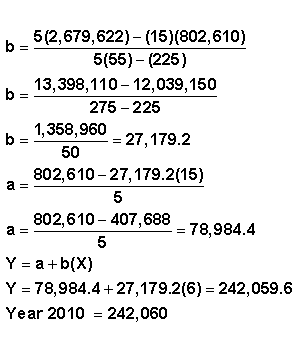Discussion

Note that all three methods produced different results. Why?

The first projection method, which basically used a ruler, relied on information primarily from the last two census periods. If growth is expected to follow the last two census takings, this method may provide a useful projection.

In the second method, the linear equation produced lower results. The data did not match the model assumptions, and the census data did not have a pattern of equal population growth for each census taking, as indicated in Figure 6-3.

The lowest projection figure of 242,060 was produced using the last method, linear regression. The equation attempted to fit a line through the data points beginning with the year 1960. It is difficult to accept the results of this projection. Durham County grew by 41,479 people between the censuses of 1990 and 2000. The projection suggests that the county will not have a similar growth pattern and will grow by less than 20,000 residents between 2000 and 2010.

Various types of economic activities in the county were investigated to understand how it has changed over the years. The county is losing jobs in an old industry and gaining employment in an area of the economy that has long-term growth prospects. People are moving into the area to take advantage of new jobs.

Based on this analysis, an average of two linear models was obtained. The ruler method was selected because the projection is based on the recent past. A linear regression analysis with a census start date of 1970 was also selected. The new regression analysis produced a projection of 247,891. The average of the two methods produced a future population size for the year 2010 of 257,279.

Question 6-1
How could a 5-year projection be calculated?

#### The Exponential Model

The exponential curve displays a pattern of constant, rapid population growth. It is an arched upward curve that has no upper limit.

Assumption
Population is assumed to grow at a geometric rate; i.e., with each unit of time, the absolute addition of population continues to get larger and larger, as shown in Figure 6-4.
When to Use
This method is suitable for short-term projections of 5–10 years for rapidly growing regions. Note that it can produce unrealistically high projections over longer periods of time.

Figure 6-4
Example of an Exponential Curve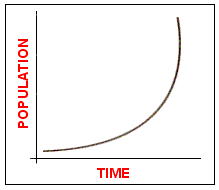The equation of the Figure 6-4 is shown in Equation 6-3.

Equation 6-3
The Exponential Equation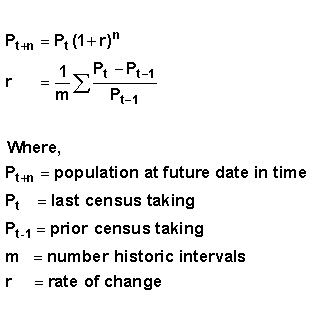The following shows an example of an exponential curve using census information for the Greater Accra Region of Ghana. If the curve is exponential, i.e., it curves upward, there are four options for calculating the population size for year 2010.

• Use Equation 6-3.
• Use logs to transform the data in order to use the linear equation.
• Transform the data using logs and use linear regression.

Question 6-2
What fourth approach could be used?

Using the Exponential Method

Examine the shape of the curve, as shown in figure 6-5.

Figure 6-5
Population Trend of Greater Accra Region, Ghana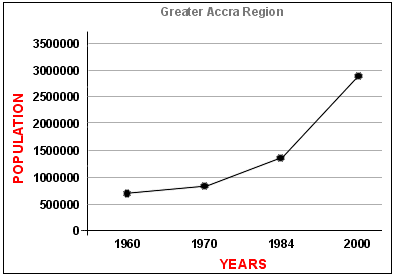Year Census of Greater Accra Region. Ghana 1960 541,933 1970 851,614 1984 1,431,100 2000 2,909,643

The pattern of the curve appears to be exponential. Next, apply the exponential equation using the data in Figure 6-5.

Exponential Approach 1: Use of the Exponential Equation
Year 2010 = 5,125,627

The first approach uses the following exponential equation to estimate r, the rate of change, by calculating increases for each time period.

Example of Equation 6-3
The Exponential Equation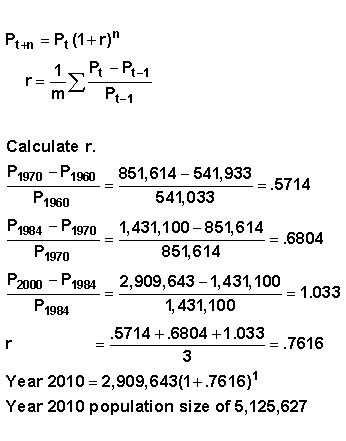Exponential Approach 2: Use of Logarithms to the Base 10 and Linear Regression: Year 2010 = 4,677,351

Logarithms can transfer an exponential growth pattern into a linear pattern of growth so that the linear equation or linear regression can be used. Log transformations translate the differences between census takings into equal change. Once the census takings represent equal or near equal change, the pattern of growth is transformed into a straight line. Table 6-2 presents log transformations of the census information for the Greater Accra Region.

 Table 6-2Logs to the Base 10 Applied to Census Data for the Greater Accra Region, Ghana Year X(year) Population Y(logs) X*Y X2 1960 1 541,933 5.733946 5.733946 1 1970 2 851,614 5.930243 11.86049 4 1984 3 1,431,000 6.15567 18.46701 9 2000 4 2,909,463 6.46384 25.85536 16 2010 Sums Sum of X  = 10 Sum of Y  =  24.2837 Sum of X*Y  =  61.9168 Sum of X2  = 30

Now, apply the linear regression equation (Y = a + bX) to the results in Table 6-2.

Application of Equation 6-2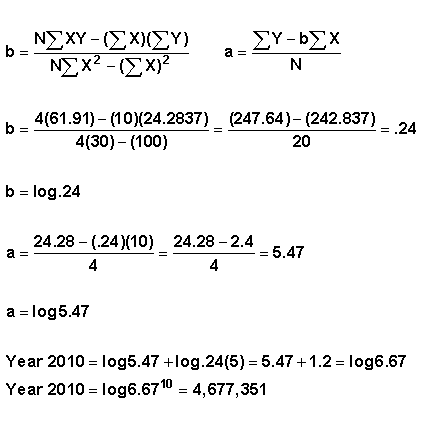Remember, X is the year of the projection. In this example, it is 5, the fifth time period.
Year 2010 = log 6.67 raised to the 10th power = 4,677,351

The results from log transformations and the use of linear regression produced lower results than the exponential equation. Log transformations dampen the effects of higher numbers relative to lower ones. Note that linear regression finds a line through all of the data points, including those years that do not reflect current growth. This may be one reason that this figure is lower than the projected population size using the other two tools.

Exponential Approach 3: Use of the Linear Equation with Log Transformations
Year 2010 = 5,011,872

The last example uses log transformations and the linear equation.

Application of Equation 6-1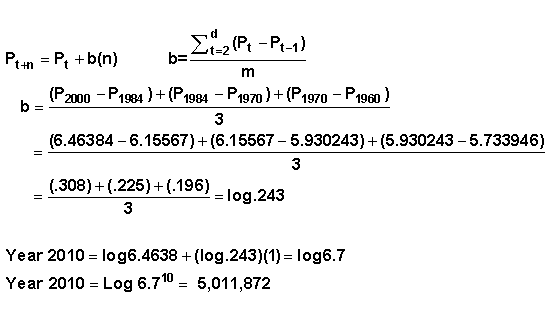Each approach produced different results. It is important to review the basis assumptions of each approach to make sure they match the information employed. Trends in economic activities and demographic behavior should also be evaluated to determine which method fits a reasonable vision of the future of the locale. Examine whether there is available land for growth in the area. Additional information is essential to select methods that will produce the best results for averaging.

#### Modified Exponential Model

A graph of the modified exponential curve is shown in Figure 6-6. This model assumes rapid population growth during an earlier point in time and slower growth in later years.

Figure 6-6
Example of a Modified Exponential Curve and Associated Data from a Rural District Census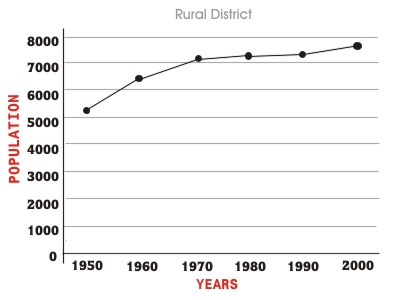Year Population 1950 5,032 1960 6,432 1970 7,022 1980 7,217 1990 7,340 2000 7,590
Assumption
Population growth reaches an upper limit in the future, at line K.
When to Use this Model
Use this model in locales that previously experienced high growth rates and are currently experiencing growth at a slower pace.

The equation for the modified exponential model is shown in Equation 6-4.

Equation 6-4
Modified Exponential Equation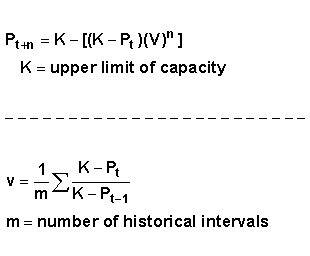The upper limit K is determined by the data. An easy but imprecise way to find K is to plot census takings on graph paper and select a point where the population levels off. For an explanation of more advanced extrapolation methods, including methods of calculating K, see Applied General Statistics (Croxton, Cowden, and Klein, 1967).

An example of the modified exponential curve is provided below. The example is based on the data in Figure 6-6. The upper limit for K is set at 8,000 residents. It is assumed that population growth is slow as a result of declining economic opportunities in the locale.

Application of Equation 6-4
Modified Exponential Equation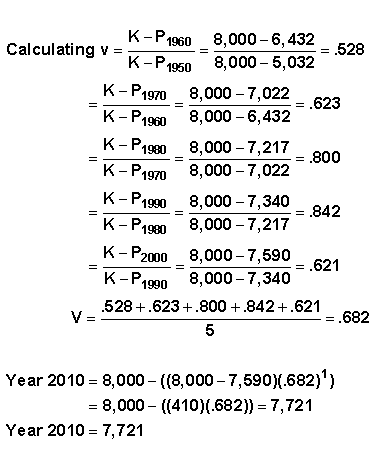To accept this projection, it is necessary to study the economy of the area as well as the carrying capacity of the land to determine if the estimate is too low or high. It may also be necessary to study the components of demographic change — migration, fertility, and mortality. For rural districts, in particular, out-migration can have a major impact on population size. Determine why growth is slow. Do young adults leave as soon as they obtain an education? Examine development plans for the district. Will new proposed activities have an impact on population growth?

Extrapolation Summary

It is important to identify the projection tool that fits the available information and provides the most reasonable projection of the future. It is a difficult task that requires investigating various tools as well as the economic and demographic behavior of the locale.

The following suggestions can aid in the selection and application of appropriate tools.

• Plot projection results on a graph and then determine if the plot best fits the observed data.
• Use a different method that fits the assumptions of the data and perform another projection. Do strong differences exist? If so, determine why. Is it the data used or the tools employed? Were there enough census takings? Was a good start date selected for the first census period?
• Check the basic assumptions of each method employed. Do the data support the assumptions?
• Examine the social, economic, and demographic trends that are taking place. Will the trends continue in the future? Do they support the projection?

### 6.2 Prorating Projection Tools

This section presents two simple prorating projection tools: the ratio and the apportionment method. Both methods rely on the projection of a larger or parent population to project the population size of a sub-area.

#### The Ratio Method

The ratio method projects population growth for a sub-area using population projections for a larger or parent population. A regional projection can be used to project the population size of districts, and a projection for the country can be used to project the population size of a region.

Assumption
Local population change is highly dependent on what happens to the population in the surrounding regions or states. The ratio method can be used to project population growth for the local area if two conditions are met: similar population patterns exist for both the locale and the parent population; and it is expected to continue in the future.
The ratio method also assumes that a projection exists for the larger or parent population. As indicated in Lesson 5, projections for large areas such as a country, province, or region tend to be more reliable than those produced for smaller locales.
When to Use this Method
Use this method for projecting the total population size for 5–10+ year periods. The ratio method summary equation is shown in Equation 6-5.

Equation 6-5
The Ratio Method Summary Equation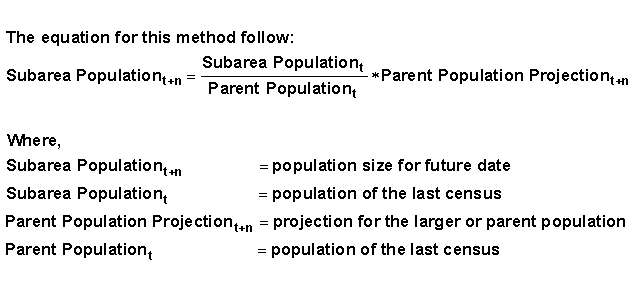How to Use this Tool:

1. Use historic census data to graph the sub-area population and the parent or state population to see if similar growth is taking place.
2. Collect projections for the parent population. Examine differences in the projections and determine why the differences exist. What methods were used and what were the assumptions?

The following example projects the total population of a region using the projection for a country. A projection of a state or province could also be used as the basis to project the size of a district. Once district projections are available, it is possible to project the size of a small town or village, provided that growth patterns are similar.

An example of the ratio method follows using a projection for Ghana in year 2010 to project the population size of the Volta Region. Ideally, a projection produced by the Ghana Statistical Service would be used. However, Ghana has only recently released their year 2000 census results, hence projections for the year 2010 were not available. This projection was performed by the International Programs Center (IPC), which is a part of the Population Division of the United States Bureau of the Census. The projection was easy to obtain via its International Data Base page. Projections produced by other international organizations such as the World Bank could have also have been used.

Projection for the Volta Region of Ghana for Year 2010
The Process of Using the Ratio Method

Step 1: First, check to see if similar growth patterns exist among the sub-area and the parent population. Figures 6-7 and 6-8 display population trends for the region and the country.

Figure 6-7
Population Trend of the Volta Region of Ghana, 1960 to 2000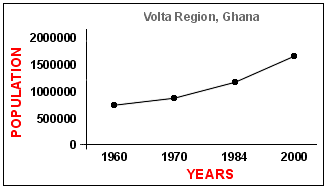Figure 6-8
Population Trend of Ghana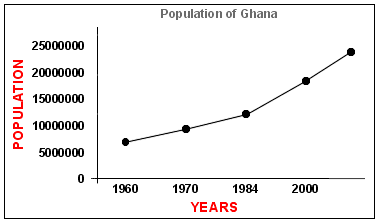Are the trends similar? Will the Volta Region continue to have similar patterns of growth? You will have to make a judgment when using this tool. In this case, the patterns of growth are similar but not identical.

Step 2: Next, obtain a reliable projection for the larger population, in this case, Ghana. The projected size of the country for year 2010 is 22,650,000. An application of the ratio method summary equation is shown below.

Application of the Ratio Method Equation, Equation 6-5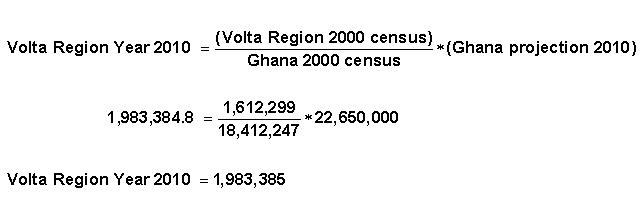The ratio tool has a number of advantages over extrapolation techniques. Computation is fast; it only takes a few minutes once the data and a reliable projection are available. Revisions are simple, and the ratio tool can be used to make long-range projections. There are, however, some disadvantages. First, like extrapolation tools, the ratio tool does not support the study of changes in births, deaths, and migration. In addition, it requires a reliable projection for the larger area. Finally, it is highly dependent on the assumed relationship between the sub-area and the larger or parent population.

How Can the Projection Results Be Improved?

The ratio technique is based on a single point in time when the calculation is performed. Calculate the ratio method for several points in time using the information for the parent and sub-area population. Once these calculations have been performed, graph the ratios and use an extrapolation tool to fit the points to a curve. This approach allows the use of more census information to determine future growth. It also allows for observation of expected population growth trends graphically.

It is possible to apply an adjustment factor to make sure that the projection represents its share of the projected growth of the region or parent population. When a number of projections are performed for a region, the sum of all sub-area projections can be much higher than the projection for the parent projection. The adjustment factor, as shown in Equation 6-6 brings all of the projections back to the projection of the parent population.

Equation 6-6
The Adjustment FactorSteps for Using the Adjustment Factor

1. Apply the ratio method for all sub-areas within the boundaries of the parent population. For example, use the ratio method for all regions if the parent population is the country, or for all districts if the parent population is a province, state, or other type of political boundary.
2. Find the proportion of the projected sub-area of the parent population.
3. Sum all of the proportions of all sub-areas
4. Divide the sum of the proportions by 1.
5. Once the adjustment factor has been calculated, multiply it by the initial projection of the sub-areas.

The following example demonstrates how to develop and apply an adjustment factor. This example uses a regional projection to project the total population size of districts.

Year 2010 Region Projection: 800,000
Year 2000 Region Census Data: 680,000

 Census for 2000 Projection 2010 Proportion Adjusted Projection District A 100,000 117,647 .147 115,908 District B 200,000 235,294 .294 231,816 District C 100,000 117,294 .147 115,908 District D 200,000 235,294 .294 231,816 District E 80,000 106,667 .133 105,090

The Process:

1. Apply the ratio technique to compute projected population size for year 2010 for District A and all other districts as shown below.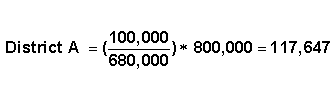2. Next, find the proportion contribution of Region A to the region's projection.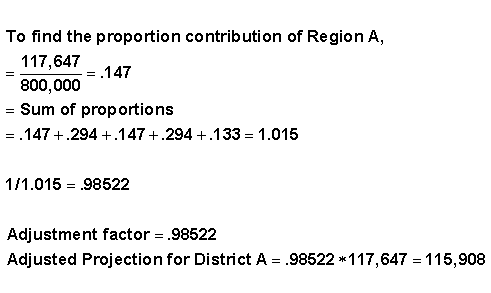The projections were adjusted to force them to equal or closely equal the region's projected total population size.

#### The Apportionment Method

The apportionment method projects the sub-area's share of the population change relative to that experienced by the larger area. The method deals with change in population as the unit of analysis, and is based on the sub-area's contribution to the historical shares of the larger area's growth.

Requirement
The distance that is being projected into the future must rely on a historical period of equal length. For example, a projection from year 2000 to 2010 must include as its historic base an interval of 10 years, beginning with 1990 to 2000.
When to Use this Method
Use the apportionment method for areas experiencing population decline or slow growth. It can also be used when the growth pattern is not similar to that of the parent population or for short- and long-term projections.

Question 6-3
What other tools can be used to do projections for declining locales?

Two steps are involved in performing this calculation. First, determine the number of people that will contribute to the growth of the parent population. Next, add that contribution to the most recent population size of the locale. These two steps are described in more detail later.

1. Determine the contribution to parent growth2. Add the contribution to the most recent census dataThe following example on the Volta Region of Ghana demonstrates how to use the tool.

 Year Ghana Change inGhana Population Volta Region Change inVolta Population Year 2010 22,650,000 Change 4,237,753 Year 2000 18,412,247 1,612,299 Change 6,116,166 400,392 Year 1984 12,296,081 1,211,907

Step 1: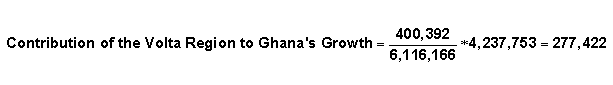Step 2: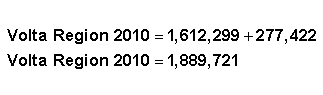Summary of Prorating Tools

Prorating tools allow planners to quickly perform a projection using both recent census information and a reliable projection of a parent population. The two methods described in this section can provide different results. The ratio method produced a projection for the Volta Region of 1,983,385, whereas the apportionment method provided a projection of 1,889,721. The ratio method assumed that similar growth patterns exist between the sub-area and parent population. Population behavior was similar for the region and the country. However, the country appears to be growing at a slightly faster pace. The apportionment method produced a lower projection. The method may not have met the assumptions of the tool.

Which Method Is Best?

Both projections could be improved by using an adjustment factor and extrapolation tools with past census figures. It is also possible to compare the results with an extrapolation tool. To do this, it would be necessary to collect additional information on the economic and demographic behaviors of the region to see which projection matches judgments about the future of the region.

### 6.3 Conclusion

These simple projection tools can assist in projecting the total population size of an area. They are simple to use and require limited information. There are a few disadvantages, however, in employing these tools. First, they do not distinguish the components of population change separately. The results of the projections don't give a sense of which demographic characteristics, fertility, mortality, or migration, contribute to population growth or decline. The extrapolation tools in particular assume that past trends will continue into the future. However, the economy or nature can influence future demographic behavior. In some cases, it is hard to accept the basic assumptions of the tools. Finally, it is important to know more about the age and sex distribution of the population to meet the needs of different segments of the population.

When using these tools, carefully analyze prior and present economic activities and demographic behavior to determine whether the projection matches future growth trends.

Exercise
Examine the trend presented in Figure 6-9. What type of extrapolation method should be applied to the growth pattern?

Figure 6-9
Population Trend of Greater Accra Region, Ghana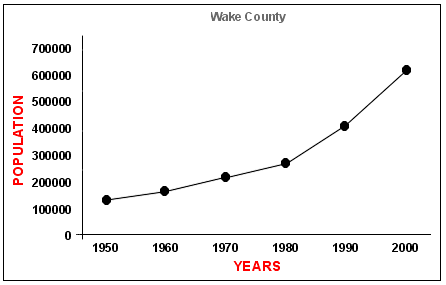Year Population 1950 136,450 1960 169,082 1970 228,453 1980 301,327 1990 423,380 2000 627,846

### Answers to Exercises

Answer — Question 6-1

Use a ruler and extend the line for 5 years versus 10 years. With regression analysis, perform the calculation for 10 years, draw the line for 10 years, and then locate 5 years on the graph

Answer — Question 6-2

There is a fourth way to project an exponential curve that is not demonstrated in this lesson. Plot the data on semi-log paper and use a ruler to find the population size for the projection period.

Answer — Question 6-3

All of the extrapolation tools. When examining a pattern of population behavior for a locale, note that the pattern can go up or down. Apply a model that reflects the pattern. A linear pattern, for example, can reveal a downward straight line.

Answer — Final Exercise 6-1

This growth pattern is exponential. There are four possible methods for calculating a projection.

1. Use the equation for exponential growth.
2. Transform the data to log base 10 and use the linear equation.
3. Transform the data to log base 10 and use linear regression.
4. Plot the data on semi-log paper and use a ruler to find the population size for the projection period.

### References

Frederick E. Croxton, Dudley J. Cowden and Sidney Klein, Applied General Statistics (Englewood Cliffs, NJ: Prentice-Hall, 1967).

Donald A. Krueckeberg and A. L. Silvers, "Projecting Population," Urban Planning Analysis: Methods and Models (New York: Wiley, 1974) 259–282.

Donald B. Pittenger, Projecting State and Local Populations (Cambridge: Ballinger, 1976).

Filed under: